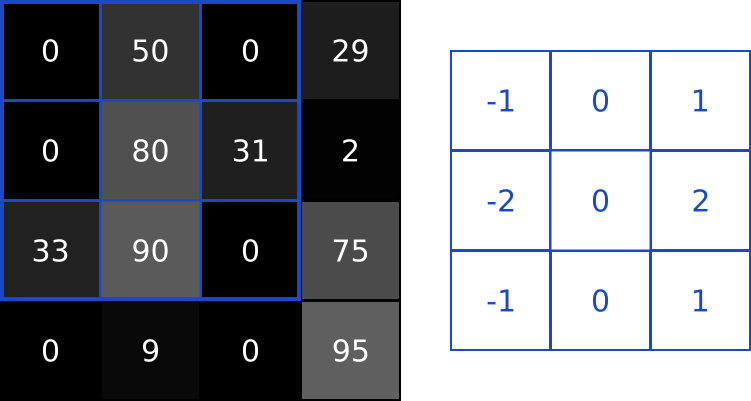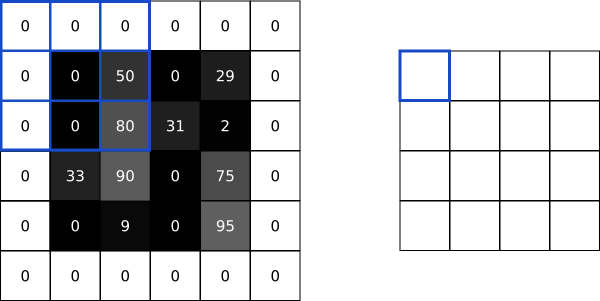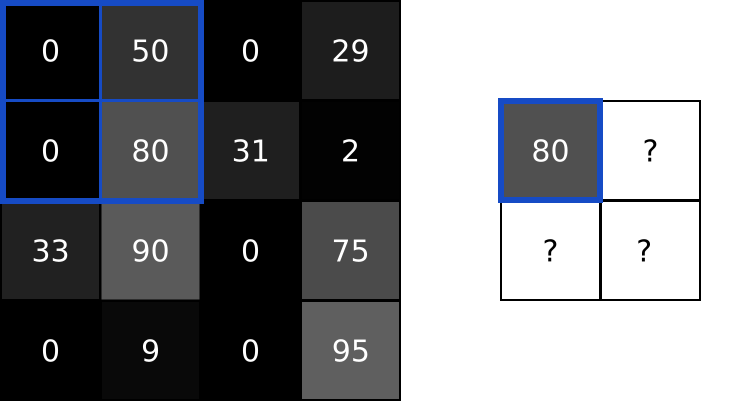An Introduction to Convolutional Neural Networks

A simple guide to what CNNs are, how they work, and how to build one from scratch in Python.

There’s been a lot of buzz about Convolution Neural Networks (CNNs) in the past few years, especially because of how they’ve revolutionized the field of Computer Vision. In this post, we’ll build on a basic background knowledge of neural networks and explore what CNNs are, understand how they work, and build a real one from scratch (using only numpy) in Python.

This post assumes only a basic knowledge of neural networks. My introduction to Neural Networks covers everything you’ll need to know, so you might want to read that first.

The formatting in this article looks best in the original post on victorzhou.com.

1. Motivation

A classic use case of CNNs is to perform image classification, e.g. looking at an image of a pet and deciding whether it’s a cat or a dog. It’s a seemingly simple task — why not just use a normal Neural Network?

Good question.

Reason 1: Images are Big

Images used for Computer Vision problems nowadays are often 224x224 or larger. Imagine building a neural network to process 224x224 color images: including the 3 color channels (RGB) in the image, that comes out to 224 x 224 x 3 = 150,528 input features! A typical hidden layer in such a network might have 1024 nodes, so we’d have to train 150,528 x 1024 = 150+ million weights for the first layer alone. Our network would be huge and nearly impossible to train.

It’s not like we need that many weights, either. The nice thing about images is that we know pixels are most useful in the context of their neighbors. Objects in images are made up of small, localized features, like the circular iris of an eye or the square corner of a piece of paper. Doesn’t it seem wasteful for every node in the first hidden layer to look at every pixel?

Reason 2: Positions can change

If you trained a network to detect dogs, you’d want it to be able to a detect a dog regardless of where it appears in the image. Imagine training a network that works well on a certain dog image, but then feeding it a slightly shifted version of the same image. The dog would not activate the same neurons, so the network would react completely differently!

We’ll see soon how a CNN can help us mitigate these problems.

2. Dataset

In this post, we’ll tackle the “Hello, World!” of Computer Vision: the MNIST handwritten digit classification problem. It’s simple: given an image, classify it as a digit.

Each image in the MNIST dataset is 28x28 and contains a centered, grayscale digit.

Truth be told, a normal neural network would actually work just fine for this problem. You could treat each image as a 28 x 28 = 784-dimensional vector, feed that to a 784-dim input layer, stack a few hidden layers, and finish with an output layer of 10 nodes, 1 for each digit.

This would only work because the MNIST dataset contains small images that are centered, so we wouldn’t run into the aforementioned issues of size or shifting. Keep in mind throughout the course of this post, however, that most real-world image classification problems aren’t this easy.

Enough buildup. Let’s get into CNNs!

3. Convolutions

What are Convolutional Neural Networks?

They’re basically just neural networks that use Convolutional layers, a.k.a. Conv layers, which are based on the mathematical operation of convolution. Conv layers consist of a set of filters, which you can think of as just 2d matrices of numbers. Here’s an example 3x3 filter:

We can use an input image and a filter to produce an output image by convolving the filter with the input image. This consists of

1. Overlaying the filter on top of the image at some location.
2. Performing element-wise multiplication between the values in the filter and their corresponding values in the image.
3. Summing up all the element-wise products. This sum is the output value for the destination pixel in the output image.
4. Repeating for all locations.
Side Note: We (along with many CNN implementations) are technically actually using cross-correlation instead of convolution here, but they do almost the same thing. I won’t go into the difference in this post because it’s not that important, but feel free to look this up if you’re curious.

That 4-step description was a little abstract, so let’s do an example. Consider this tiny 4x4 grayscale image and this 3x3 filter:

The numbers in the image represent pixel intensities, where 0 is black and 255 is white. We’ll convolve the input image and the filter to produce a 2x2 output image:

To start, lets overlay our filter in the top left corner of the image:Step 1: Overlay the filter (right) on top of the image (left)

Next, we perform element-wise multiplication between the overlapping image values and filter values. Here are the results, starting from the top left corner and going right, then down:

Next, we sum up all the results. That’s easy enough: 62–33=29.

Finally, we place our result in the destination pixel of our output image. Since our filter is overlayed in the top left corner of the input image, our destination pixel is the top left pixel of the output image:

We do the same thing to generate the rest of the output image:

3.1 How is this useful?

Let’s zoom out for a second and see this at a higher level. What does convolving an image with a filter do? We can start by using the example 3x3 filter we’ve been using, which is commonly known as the vertical Sobel filter:

Here’s an example of what the vertical Sobel filter does:

Similarly, there’s also a horizontal Sobel filter:

See what’s happening? Sobel filters are edge-detectors. The vertical Sobel filter detects vertical edges, and the horizontal Sobel filter detects horizontal edges. The output images are now easily interpreted: a bright pixel (one that has a high value) in the output image indicates that there’s a strong edge around there in the original image.

Can you see why an edge-detected image might be more useful than the raw image? Think back to our MNIST handwritten digit classification problem for a second. A CNN trained on MNIST might look for the digit 1, for example, by using an edge-detection filter and checking for two prominent vertical edges near the center of the image. In general, convolution helps us look for specific localized image features (like edges) that we can use later in the network.

Remember convolving a 4x4 input image with a 3x3 filter earlier to produce a 2x2 output image? Often times, we’d prefer to have the output image be the same size as the input image. To do this, we add zeros around the image so we can overlay the filter in more places. A 3x3 filter requires 1 pixel of padding:A 4x4 input convolved with a 3x3 filter to produce a 4x4 output using same padding

This is called “same” padding, since the input and output have the same dimensions. Not using any padding, which is what we’ve been doing and will continue to do for this post, is sometimes referred to as “valid” padding.

3.3 Conv Layers

Now that we know how image convolution works and why it’s useful, let’s see how it’s actually used in CNNs. As mentioned before, CNNs include conv layers that use a set of filters to turn input images into output images. A conv layer’s primary parameter is the number of filters it has.

For our MNIST CNN, we’ll use a small conv layer with 8 filters as the initial layer in our network. This means it’ll turn the 28x28 input image into a 26x26x8 output volume:

Reminder: The output is 26x26x8 and not 28x28x8 because we’re using valid padding, which decreases the input’s width and height by 2.

Each of the 8 filters in the conv layer produces a 26x26 output, so stacked together they make up a 26x26x8 volume. All of this happens because of 3 x 3 (filter size) x 8 (number of filters) = only 72 weights!

3.4 Implementing Convolution

Time to put what we’ve learned into code! We’ll implement a conv layer’s feedforward portion, which takes care of convolving filters with an input image to produce an output volume. For simplicity, we’ll assume filters are always 3x3 (which is not true — 5x5 and 7x7 filters are also very common).

Let’s start implementing a conv layer class:

The `Conv3x3` class takes only one argument: the number of filters. In the constructor, we store the number of filters and initialize a random filters array using NumPy's randn() method.

Note: Diving by 9 during the initialization is more important than you may think. If the initial values are too large or too small, training the network will be ineffective. To learn more, read about Xavier Initialization.

Next, the actual convolution:

`iterate_regions()`is a helper generator method that yields all valid 3x3 image regions for us. This will be useful for implementing the backwards portion of this class later on.

Line 26 actually performs the convolutions. Let’s break it down:

• We have `im_region`, a 3x3 array containing the relevant image region.
• We have `self.filters`, a 3d array.
• We do , which uses numpy’s broadcasting feature to element-wise multiply the two arrays. The result is a 3d array with the same dimension as `self.filters`.
• We np.sum() the result of the previous step using , which produces a 1d array of length `num_filters` where each element contains the convolution result for the corresponding filter.

The sequence above is performed for each pixel in the output until we obtain our final output volume! Let’s give our code a test run:

Looks good so far.

Note: in our `Conv3x3` implementation, we assume the input is a 2d numpy array for simplicity, because that's how our MNIST images are stored. This works for us because we use it as the first layer in our network, but most CNNs have many more Conv layers. If we were building a bigger network that needed to use `Conv3x3` multiple times, we'd have to make the input be a 3d numpy array.

4. Pooling

Neighboring pixels in images tend to have similar values, so conv layers will typically also produce similar values for neighboring pixels in outputs. As a result, much of the information contained in a conv layer’s output is redundant. For example, if we use an edge-detecting filter and find a strong edge at a certain location, chances are that we’ll also find relatively strong edges at locations 1 pixel shifted from the original one. However, these are all the same edge! We’re not finding anything new.

Pooling layers solve this problem. All they do is reduce the size of the input it’s given by (you guessed it) pooling values together in the input. The pooling is usually done by a simple operation like `max`, `min`, or `average`. Here's an example of a Max Pooling layer with a pooling size of 2:Max Pooling (pool size 2) on a 4x4 image to produce a 2x2 output

To perform max pooling, we traverse the input image in 2x2 blocks (because pool size = 2) and put the max value into the output image at the corresponding pixel. That’s it!

Pooling divides the input’s width and height by the pool size. For our MNIST CNN, we’ll place a Max Pooling layer with a pool size of 2 right after our initial conv layer. The pooling layer will transform a 26x26x8 input into a 13x13x8 output:

4.1 Implementing Pooling

We’ll implement a `MaxPool2` class with the same methods as our conv class from the previous section:

This class works similarly to the `Conv3x3` class we implemented previously. The critical line is line 30: to find the max from a given image region, we use np.amax(), numpy's array max method. We set because we only want to maximize over the first two dimensions, height and width, and not the third, `num_filters`.

Let’s test it!

Our MNIST CNN is starting to come together!

5. Softmax

To complete our CNN, we need to give it the ability to actually make predictions. We’ll do that by using the standard final layer for a multiclass classification problem: the Softmax layer, a standard fully-connected (dense) layer that uses the softmax activation function.

Reminder: fully-connected layers have every node connected to every output from the previous layer. We used fully-connected layers in my intro to Neural Networks if you need a refresher.

Softmax turns arbitrary real values into probabilities. The math behind it is pretty simple: given some numbers,

1. Raise e (the mathematical constant) to the power of each of those numbers.
2. Sum up all the exponentials (powers of e). This result is the denominator.
3. Use each number’s exponential as its numerator.
4. Probability = Numerator / Denominator

Written more fancily, Softmax performs the following transform on n numbers x1, … xn:

The outputs of the Softmax transform are always in the range [0, 1] and add up to 1. Hence, they’re probabilities.

Here’s a simple example using the numbers -1, 0, 3, and 5:

5.1 Usage

We’ll use a softmax layer with 10 nodes, one representing each digit, as the final layer in our CNN. Each node in the layer will be connected to every input. After the softmax transformation is applied, the digit represented by the node with the highest probability will be the output of the CNN!

5.2 Cross-Entropy Loss

You might have just thought to yourself, why bother transforming the outputs into probabilities? Won’t the highest output value always have the highest probability? If you did, you’re absolutely right. We don’t actually need to use softmax to predict a digit — we could just pick the digit with the highest output from the network!

What softmax really does is help us quantify how sure we are of our prediction, which is useful when training and evaluating our CNN. More specifically, using softmax lets us use cross-entropy loss, which takes into account how sure we are of each prediction. Here’s how we calculate cross-entropy loss:

where c is the correct class (in our case, the correct digit), pc​ is the predicted probability for class c, and ln is the natural log. As always, a lower loss is better. For example, in the best case, we’d have

In a more realistic case, we might have

We’ll be seeing cross-entropy loss again later on in this post, so keep it in mind!

5.3 Implementing Softmax

You know the drill by now — let’s implement a `Softmax` layer class:

There’s nothing too complicated here. A few highlights:

• We flatten() the input to make it easier to work with, since we no longer need its shape.
• np.dot() multiplies `input` and `self.weights` element-wise and then sums the results.
• np.exp() calculates the exponentials used for Softmax.

We’ve now completed the entire forward pass of our CNN! Putting it together:

Running `cnn.py` gives us output similar to this:

``MNIST CNN initialized![Step 100] Past 100 steps: Average Loss 2.302 | Accuracy: 11%[Step 200] Past 100 steps: Average Loss 2.302 | Accuracy: 8%[Step 300] Past 100 steps: Average Loss 2.302 | Accuracy: 3%[Step 400] Past 100 steps: Average Loss 2.302 | Accuracy: 12%``

This makes sense: with random weight initialization, you’d expect the CNN to be only as good as random guessing. Random guessing would yield 10% accuracy (since there are 10 classes) and a cross-entropy loss of −ln(0.1)=2.302, which is what we get!

Want to try or tinker with this code yourself? Run this CNN in your browser. It’s also available on Github.

6. Conclusion

That’s the end of this introduction to CNNs! In this post, we

• Motivated why CNNs might be more useful for certain problems, like image classification.
• Introduced the MNIST handwritten digit dataset.
• Learned about Conv layers, which convolve filters with images to produce more useful outputs.
• Talked about Pooling layers, which can help prune everything but the most useful features.
• Implemented a Softmax layer so we could use cross-entropy loss.

There’s still much more that we haven’t covered yet, such as how to actually train a CNN. My next post will do a deep-dive on training a CNN, including deriving gradients and implementing backprop, so stay tuned!

If you’re eager to see a trained CNN in action: this example Keras CNN trained on MNIST achieves 99.25% accuracy. CNNs are powerful!

Originally published at https://victorzhou.com.